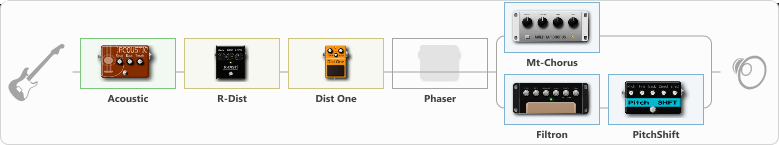# fuzz lightyear

Discussion in 'ToneLib-GFX presets' started by whiskeybentbmx, Dec 17, 2021.

1. fuzz lightyear

Preset name: fuzz lightyear

Effects chain:Effect: "Acoustic" (Dynamics / Filter), active - "yes"
{
"Style" = Slide
"Resonance" = 100
"Bass" = 100
"Treble" = 21
}

Effect: "R-Dist" (Overdrive / Distortion), active - "yes"
{
"Drive" = 74
"Tone" = 35
"Level" = 68
}

Effect: "Dist One" (Overdrive / Distortion), active - "yes"
{
"Drive" = 50
"Tone" = 100
"Level" = 69
}

Effect: "Phaser" (Modulation / Sfx), active - "no"
{
"Model" = MXR Phase 90
"Speed" = 4.0
"Depth" = 100
"Reso" = 26
"Freq" = 9.4
}

Effect: "Splitter" (Dynamics / Filter)
{
"A-Bypass" = Off
"A-Pan" = 0
"A-Level" = 55
"B-Bypass" = Off
"B-Pan" = 0
"B-Level" = 55

'A' branch:
{

Effect: "Mt-Chorus" (Modulation / Sfx), active - "yes"
{
"Speed" = 5.3
"Depth" = 92
"Time" = 2.5
"Mix" = 67
}
}
'B' branch:
{

Effect: "Filtron" (Modulation / Sfx), active - "yes"
{
"Attack" = 3.8
"Depth" = 95
"Reso" = 72
"Cutoff" = 2.5
"Polarity" = Up
"Sens" = 63
}

Effect: "PitchShift" (Modulation / Sfx), active - "yes"
{
"Pitch" = 7
"Fine" = 0
"Tracking" = 85
"Direct" = 100
"Effect" = 80
}
}
}

Note: You will need to download and install the ToneLib-GFX software to use the preset.

File size:
795 bytes
Views:
2,481